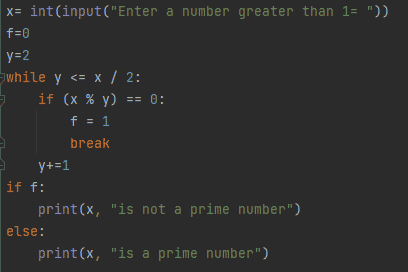Scripting

# How to Check if a Number is Prime in PythonA prime number is a number that is not divisible by any other number except one so in this article we will teach you multiple ways how you can check if the number is prime or not in python.

1. By user defined function
2. By using a flag variable
3. By using while loop

## 1. By User Defined Function

In python we can define a function using def keyword which is a good choice to define a function to determine whether the number is a prime number or no as defining function increases the usability of code

```def checkprime(x):

if x > 1:

for y in range(2, int(x/2) + 1):

if (x % y) == 0:

print(x, "is not a prime number")

break

else:

print(x, "is a prime number")

else:

print(x, "is not a prime number")

a= int(input("Enter a number = "))

checkprime(a)```Figure 1: Using nested if else

Output:Figure 2: Output

In the figure 1, first we have declared a function checkprime(a) that takes a number as parameter and checks whether the given number is bigger than one. If the number is greater than 1, for loop will be executed to check if ‘x’ is fully divisible by ‘y’ then ‘x’ is not a prime number otherwise, the ‘x’ is a prime number. If the number is not larger than 1, it will go straight to the else part and print ’x’ is not a prime number as shown in figure 2.

## 2. By using a flag variable

In Python, we can verify if a number is prime or not by using a flag variable. Flag variable can have two values: true or false.

```x= int(input("Enter a number = "))

flag = False

if x > 1:

for y in range(2, x):

if (x % y) == 0:

flag = True

break

if flag:

print(x, "is not a prime number")

else:

print(x, "is a prime number")```Figure 3: Using flag variable

Output:Figure 4: Output

In the above example, User enters a number and we check if entered number ‘x’ is more than one or not, so we only proceed when ‘x’ is more than one. If ‘x’ is divisible by any number in the range (2, x), set flag to True and exit the loop if a factor in that range is found, indicating that the integer is not prime, as illustrated in figure 2. Otherwise, the value of the flag will stay False, indicating that the number is prime.

## 3. By using while loop

Prime number can be checked using while loop.

```x= int(input("Enter a number greater than 1= "))

f=0

y=2

while y <= x / 2:

if (x % y) == 0:

f = 1

break

y+=1

if f:

print(x, "is not a prime number")

else:

print(x, "is a prime number")```

Figure 5: Using while loop

Output:Figure 6: Output

In the above example user is asked to enter a number ‘x’ greater than 1. The while loop condition is checked if it’s true then it will be executed. Firstly, remainder will be calculated to see if ‘x’ is fully divisible by any other number than itself. If ‘x’ is fully divisible then value of variable ‘f’ will be updated otherwise it will not be updated.